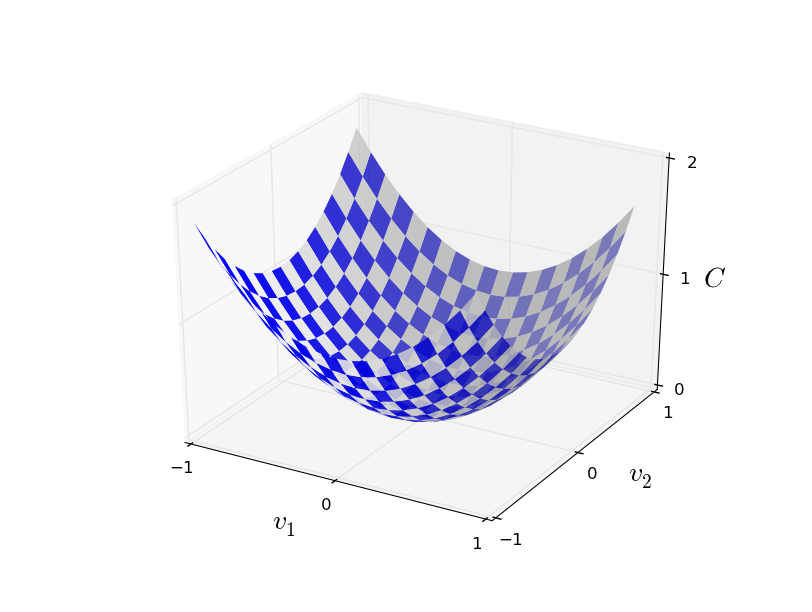# 神经网络和深度学习(1)

Categories 机器学习## 前言

This work is licensed under a Creative Commons Attribution-NonCommercial 3.0 Unported License. This means you’re free to copy, share, and build on this book, but not to sell it. If you’re interested in
commercial use, please contact me.

• 神经网络： 一个从生物学得到启发的漂亮的编程方法，让电脑可以从可观察的数据中进行学习
• 深度学习：运用神经网络机型学习的极为有效的技术体系

## 概念Fig 1. 手写字体

• 准备一系列已知的手写数据范例（如下图），称为训练数据
• 创造一个识别系统，从这些手写数据范例中归纳出识别的模式。Fig 2. 手写字体范例

### 感知器Fig 3. 神经元

$$\begin{eqnarray} \mbox{output} & = & \left\{ \begin{array}{ll} 0 & \mbox{if } \sum_j w_j x_j \leq \mbox{ threshold} \\ 1 & \mbox{if } \sum_j w_j x_j > \mbox{ threshold} \end{array} \right. \tag{1}\end{eqnarray}$$

$$\begin{eqnarray} \mbox{output} & = & \left\{ \begin{array}{ll} 0 & \mbox{if } \sum_j w_j x_j +b\leq \mbox{0} \\ 1 & \mbox{if } \sum_j w_j x_j+b > \mbox{ 0} \end{array} \right. \tag{2}\end{eqnarray}$$Fig 4. Perceptron(感知器)

### 神经网络

• 感知器：即下图每一个小圆，神经网络的“神经元”
• 输入层：由N个感知器组成的层次（N为输入条件的个数）
• 输出层：有M个感知器组成的层次，（N为机器学习问题输出值的个数。对手写数字识别来讲，即有10种可能输出，即输出层由10个感知器组成）
• 多个隐层（Hidden Layer）： 非输入层也非输出层的中间层。可以为0个或者多个。
• 层与层连线上的参数 ，即感知器的权值 $$w_{ij}$$ 和Threshold $$b$$Fig 5. Neural Network(神经网络)

$$w_{ij}$$ 与阈值 $$b$$ ，直到所有检验数据达到需要的精准度。

### Sigmoid神经元Fig 6. 函数(2)的图形

$$f\equiv\frac{1}{1+e^{-{(W \bullet X-b)}}} \tag{3}$$Fig 7. Sigmoid函数的图形

$$\begin{eqnarray} \Delta \mbox{output} \approx \sum_j \frac{\partial \, \mbox{output}}{\partial w_j} \Delta w_j + \frac{\partial \, \mbox{output}}{\partial b} \Delta b, \tag{5} \end{eqnarray}$$

## 识别手写数字实战

1. 将上述图片分割为6个独立的数字
2. 对6个独立的数字分别进行识别图片分解

• 输入层$$784=28*28$$，表示每个被分割出来的数字有28乘28个像素点组成。每个像素点值表示颜色深度：0值表示该像素位置为白色，1则表示黑色，0到1的中间数字表示介于黑白之间像素点的灰黑色深度。
• 隐层神经元个数的选择对学习效率是有影响的（事实上，如何选择这些初始值也是非常值得研究的课题）。本文从15作为初始选择，后边实例将会观察不同隐层神经元个数对学习结果的影响。
• 输出层则比较直觉，10个输出代表我们要识别的数字的10中可能。我们当然可以采用其他个数的神经元组合。例如：神经元代表每个二进制位的值，这样只需要4个神经元就能表示0~16的值。但事实上，这样的选择效果往往不会非常的理想。试想，1~10这10个数字的图片形状各不相同，如何用八个形状（4个bit*每个bit 2个值）来归纳这十个数字？

### 梯度下降法学习

• train-images-idx3-ubyte: 训练集图片
• train-labels-idx1-ubyte: 训练集标签
• t10k-images-idx3-ubyte:  测试集图片
• t10k-labels-idx1-ubyte:  测试集标签

 偏移 类型 数值 描述 0000 32位整形 0x00000803(2051 MAGIC Number（魔数） 0004 32位整形 60000 或 10000 图片个数 0008 32位整形 28 像素行数 0012 32位整形 28 像素列数 0016 无符号字节 ？ 像素点 0017 无符号字节 ？ 像素点 …….. 无符号字节 ？ 像素点 xxxx 无符号字节 ？ 像素点

 偏移 类型 数值 描述 0000 32位整形 0x00000801(2049 MAGIC Number（魔数） 0004 32位整形 60000 图片个数 0008 无符号字节 ？ 标签值（0-9） 0009 无符号字节 ？ 标签值（0-9） …….. 无符号字节 ？ 标签值（0-9） xxxx 无符号字节 ？ 标签值（0-9）

$$\begin{eqnarray} C(w,b) \equiv \frac{1}{2n} \sum_x \| y(x) – a\|^2 \nonumber\end{eqnarray}$$

$$w$$表示网络中的所有权重，$$b$$代表所有的阈值，$$n$$表示训练数据总量，$$a$$则是$$x$$作为训练数据时的输出结果向量。那么该误差函数$$C(w,b)$$表示的就是目标函数输出与实际训练数据结果的均方误差，mean squared error or just(MSE)。当目标函数与输出结果接近时，$$C(w,b) \approx 0$$。因此我们的目标便是对所有训练数据，使得目标函数结果与训练数据的输出接近（当然最好是相等，XD）。接下来就该梯度下降算法出场了。全局最小

$$\begin{eqnarray} \Delta C \approx \frac{\partial C}{\partial v_1} \Delta v_1 + \frac{\partial C}{\partial v_2} \Delta v_2. \tag{6}\end{eqnarray}$$

$$\begin{eqnarray} \nabla C \equiv \left( \frac{\partial C}{\partial v_1}, \frac{\partial C}{\partial v_2} \right)^T. \tag{7}\end{eqnarray}$$

$$\begin{eqnarray} \Delta C \approx \nabla C \cdot \Delta v. \tag{8}\end{eqnarray}$$

$$\begin{eqnarray} \Delta v = -\eta \nabla C, \tag{9}\end{eqnarray}$$

$$\begin{eqnarray} w_k & \rightarrow & w_k’ = w_k-\eta \frac{\partial C}{\partial w_k} \tag{10}\\ b_l & \rightarrow & b_l’ = b_l-\eta \frac{\partial C}{\partial b_l}. \tag{11}\end{eqnarray}$$

$$\nabla C = \frac{1}{m} \sum_x \nabla C_x$$ 。最后我们得到参数的更新公式：

$$\begin{eqnarray} w_k & \rightarrow & w_k’ = w_k-\frac{\eta}{m} \sum_j \frac{\partial C_{X_j}}{\partial w_k} \tag{12}\\ b_l & \rightarrow & b_l’ = b_l-\frac{\eta}{m} \sum_j \frac{\partial C_{X_j}}{\partial b_l}, \tag{13}\end{eqnarray}$$

## 代码实例

#### Libraries
# 标准随机库
import random

# numpy库
import numpy as np

class Network(object):

def __init__(self, sizes):
""“
sizes定义是每层神经网络的神经元（感知器）个数。例如若sizes=[2,3,1]，那么它代表输入层2个感知器，隐层3个感知器，最后一层1个感知器。
"""
self.num_layers = len(sizes)
self.sizes = sizes
#初始化每层的权值w和b（bias即负的阈值）
self.biases = [np.random.randn(y, 1) for y in sizes[1:]]
self.weights = [np.random.randn(y, x)
for x, y in zip(sizes[:-1], sizes[1:])]

def feedforward(self, a):
"""返回感知器的输出z"""
for b, w in zip(self.biases, self.weights):
a = sigmoid(np.dot(w, a)+b)
return a

def SGD(self, training_data, epochs, mini_batch_size, eta,
test_data=None):
"""使用前文提到的‘每次学习只取训练数据集的一部分m个，只要保证最后所有训练数据都有被取到且平均即可’算法进行训练"""
if test_data: n_test = len(test_data)
n = len(training_data)
#训练轮数
for j in xrange(epochs):
#随机打乱训练数据
random.shuffle(training_data)
#根据训练最小包的个数分出多个训练包
mini_batches = [
training_data[k:k+mini_batch_size]
for k in xrange(0, n, mini_batch_size)]
#对每个训练数据包进行训练，权值w和b进行更新
for mini_batch in mini_batches:
self.update_mini_batch(mini_batch, eta)
#用测试数据对训练结果进行计算准确率
if test_data:
print "Epoch {0}: {1} / {2}".format(
j, self.evaluate(test_data), n_test)
else:
print "Epoch {0} complete".format(j)

def update_mini_batch(self, mini_batch, eta):
"""使用后传播方法对训练数据包进行训练（更新w和b）"""
#初始化所有的b和w
nabla_b = [np.zeros(b.shape) for b in self.biases]
nabla_w = [np.zeros(w.shape) for w in self.weights]
#根据包中所有的训练数据计算w和b要变化的幅度nabla_b和nabla_w
for x, y in mini_batch:
delta_nabla_b, delta_nabla_w = self.backprop(x, y)
nabla_b = [nb+dnb for nb, dnb in zip(nabla_b, delta_nabla_b)]
nabla_w = [nw+dnw for nw, dnw in zip(nabla_w, delta_nabla_w)]
#根据计算的更新幅度更新w和b
self.weights = [w-(eta/len(mini_batch))*nw
for w, nw in zip(self.weights, nabla_w)]
self.biases = [b-(eta/len(mini_batch))*nb
for b, nb in zip(self.biases, nabla_b)]

def backprop(self, x, y):
nabla_b = [np.zeros(b.shape) for b in self.biases]
nabla_w = [np.zeros(w.shape) for w in self.weights]
# feedforward
activation = x
activations = [x] # list to store all the activations, layer by layer
zs = []
# 根据w和b计算训练数据输入得到每个神经元输出
for b, w in zip(self.biases, self.weights):
z = np.dot(w, activation)+b
zs.append(z)
activation = sigmoid(z)
activations.append(activation)
# 计算输出层对w和b的偏导
delta = self.cost_derivative(activations[-1], y) * \
sigmoid_prime(zs[-1])
nabla_b[-1] = delta
nabla_w[-1] = np.dot(delta, activations[-2].transpose())
# 从倒数第二层到输入层计算每层w和b的更新值，这里的为BP神经网络算法的实现方法，后续会再介绍其原理。
for l in xrange(2, self.num_layers):
z = zs[-l]
sp = sigmoid_prime(z）
delta = np.dot(self.weights[-l+1].transpose(), delta) * sp
nabla_b[-l] = delta
nabla_w[-l] = np.dot(delta, activations[-l-1].transpose())
return (nabla_b, nabla_w)

def evaluate(self, test_data):
#此处比较容易理解，使用测试数据和我们计算得到的w和b代入的公式得到结果对比计算当前学习的准确率
test_results = [(np.argmax(self.feedforward(x)), y)
for (x, y) in test_data]
return sum(int(x == y) for (x, y) in test_results)

def cost_derivative(self, output_activations, y):
return (output_activations-y)
#sigmoid函数
def sigmoid(z):
"""The sigmoid function."""
return 1.0/(1.0+np.exp(-z))
#sigmoid函数的倒数
def sigmoid_prime(z):
"""Derivative of the sigmoid function."""
return sigmoid(z)*(1-sigmoid(z))

## 参考文献

1. Neural Networks and Deep Learning